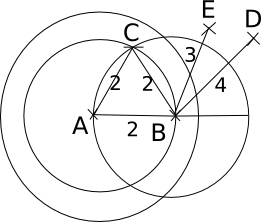### CAT 1996 Question Paper Question 9

Instructions

Direction for questions 108 and 109: Answer the questions based on the following information. In a locality, there are five small cities: A, B, C, D and E. The distances of these cities from each other are as follows. AB = 2 km AC = 2km AD > 2 km AE > 3 km BC = 2 km BD = 4 km BE = 3 km CD = 2 km CE = 3 km DE > 3 km

Question 9

# If a ration shop is to be set up within 3 km of each city, how many ration shops will be required?

Solution

Consider the following scenario:So, if a ration shop is setup on the line joining A and E, just inside the 3 km radius circle, it will be within 3 km of each city.